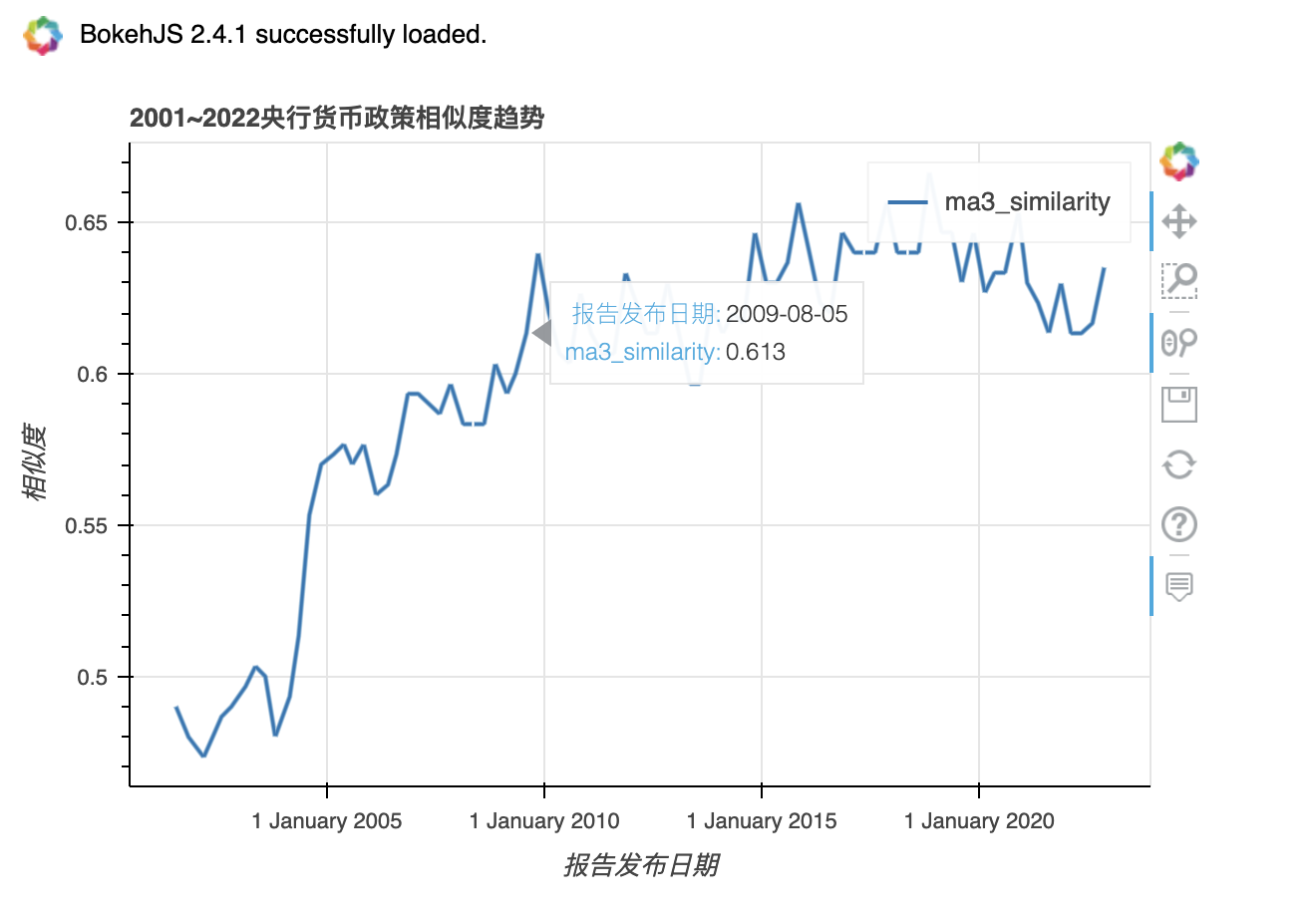title: 金融研究 | 央行货币政策文本相似度计算与可视化
author: "大邓"
date: '2023-01-10'
tags:
- 文本分析
- 经济管理
cover:
image: images/blog/simi_finance_cover_paper.png
description: 本文利用金融情感词典和文本分析技术,分析中国人民银行货币政策执行报告的**文本情绪、文本相似度和文本可读性**等多维文本信息,刻画央行货币政策执行报告的文本特征,探究货币政策报告的文本信息与宏观经济和股票市场的关系。**实证研究发现,货币政策报告的文本情绪的改善会引起显著为正的股票市场价格反应, 报告文本相似度的增加会引起股票市场波动性的显著降低, 报告可读性对公布后股票市场的波动性影响不显著**。货币政策报告文本情绪还与诸多宏观经济指标显著相关。进一步研究发现,引起股票市场显著反应的是报告文本情绪中反映货币政策指引的部分,而反映宏观经济历史状态的部分对股票市场的影响不显著。本文从文本大数据分析角度证明了我国央行沟通的有效性,对国内央行沟通相关研究形成了有益补充。
keywords:
- 文本相似度
- 金融研究
- 央行货币政策
draft: no
type: post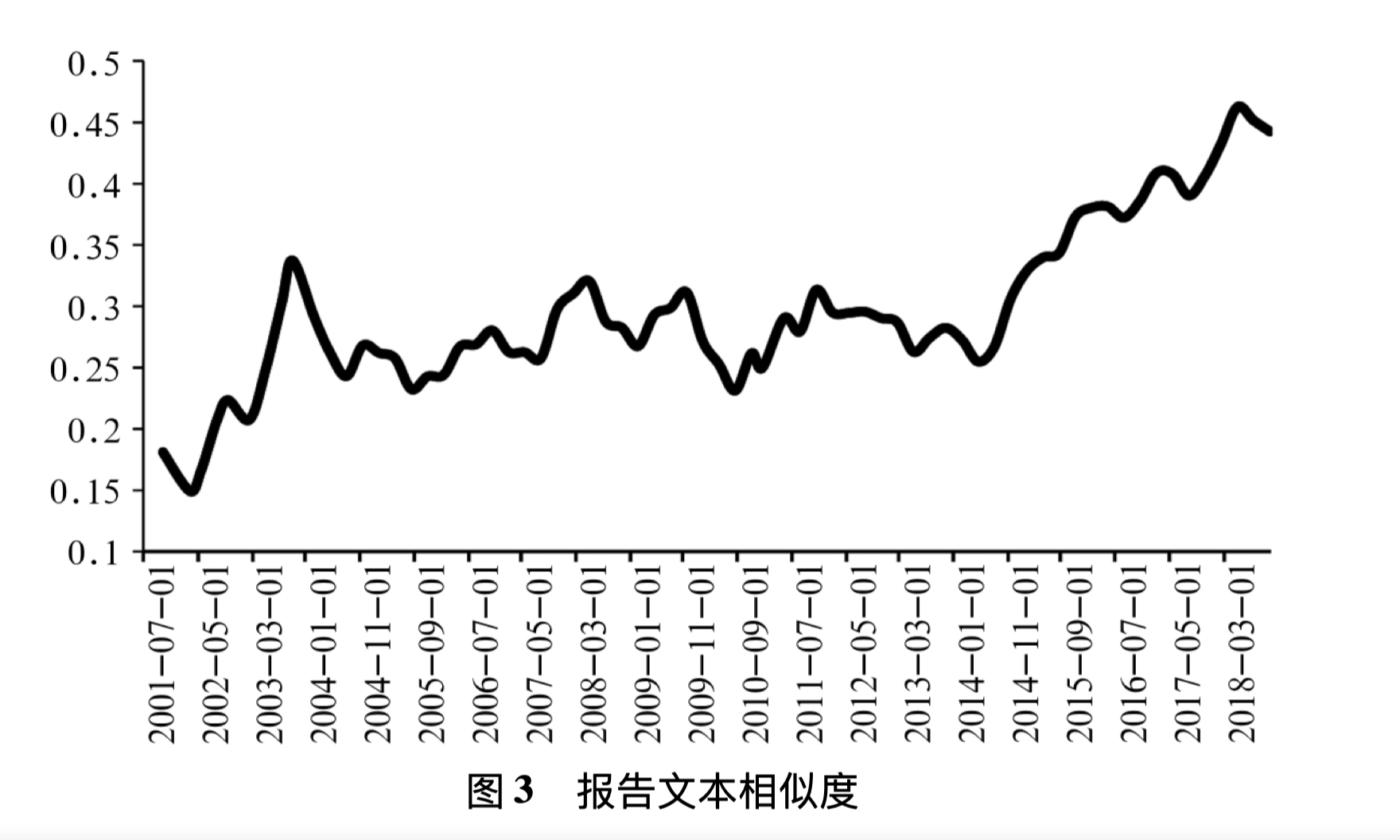1. 准备数据
2. 相似度计算
3. 可视化

## 1. 准备数据#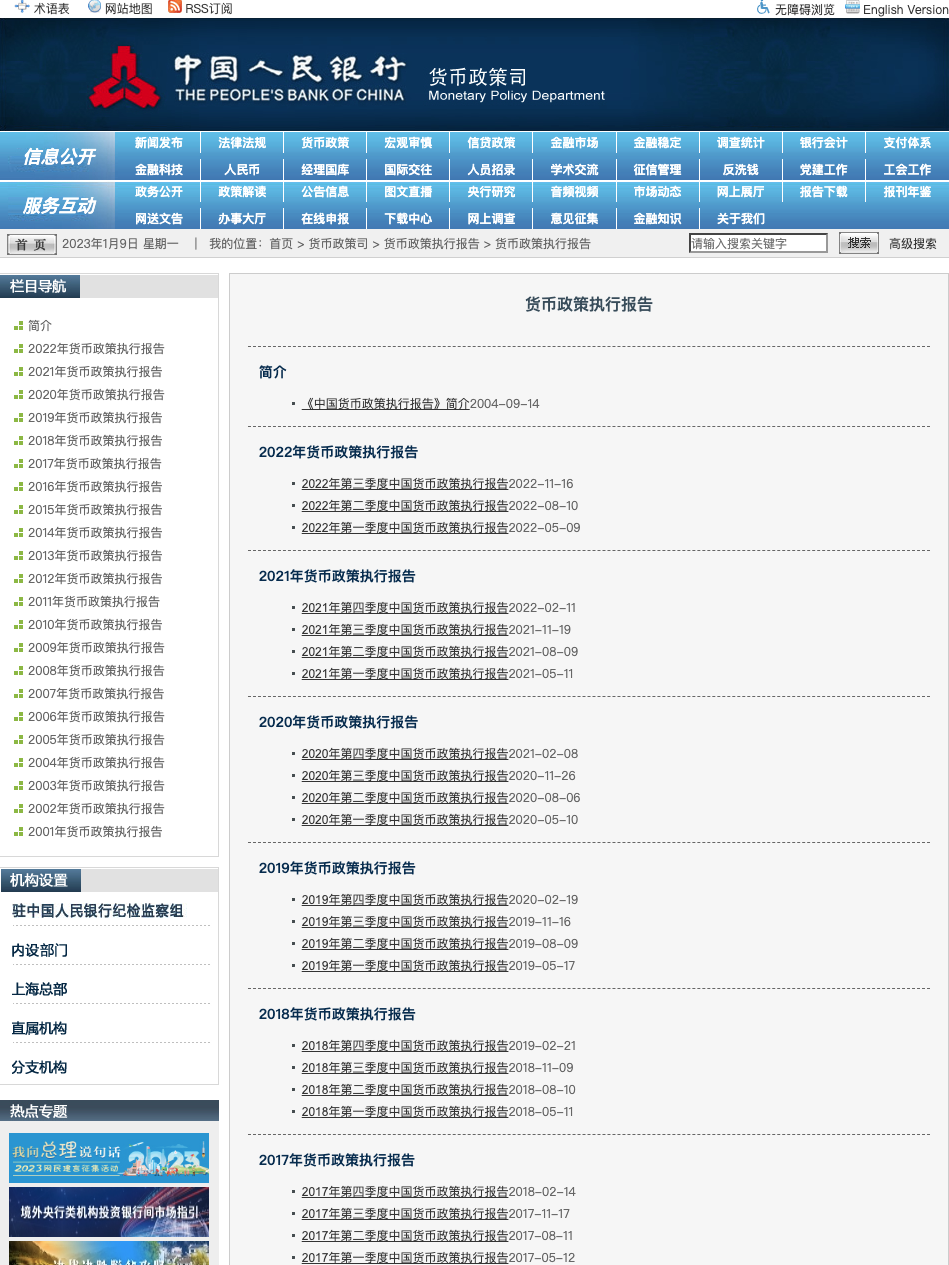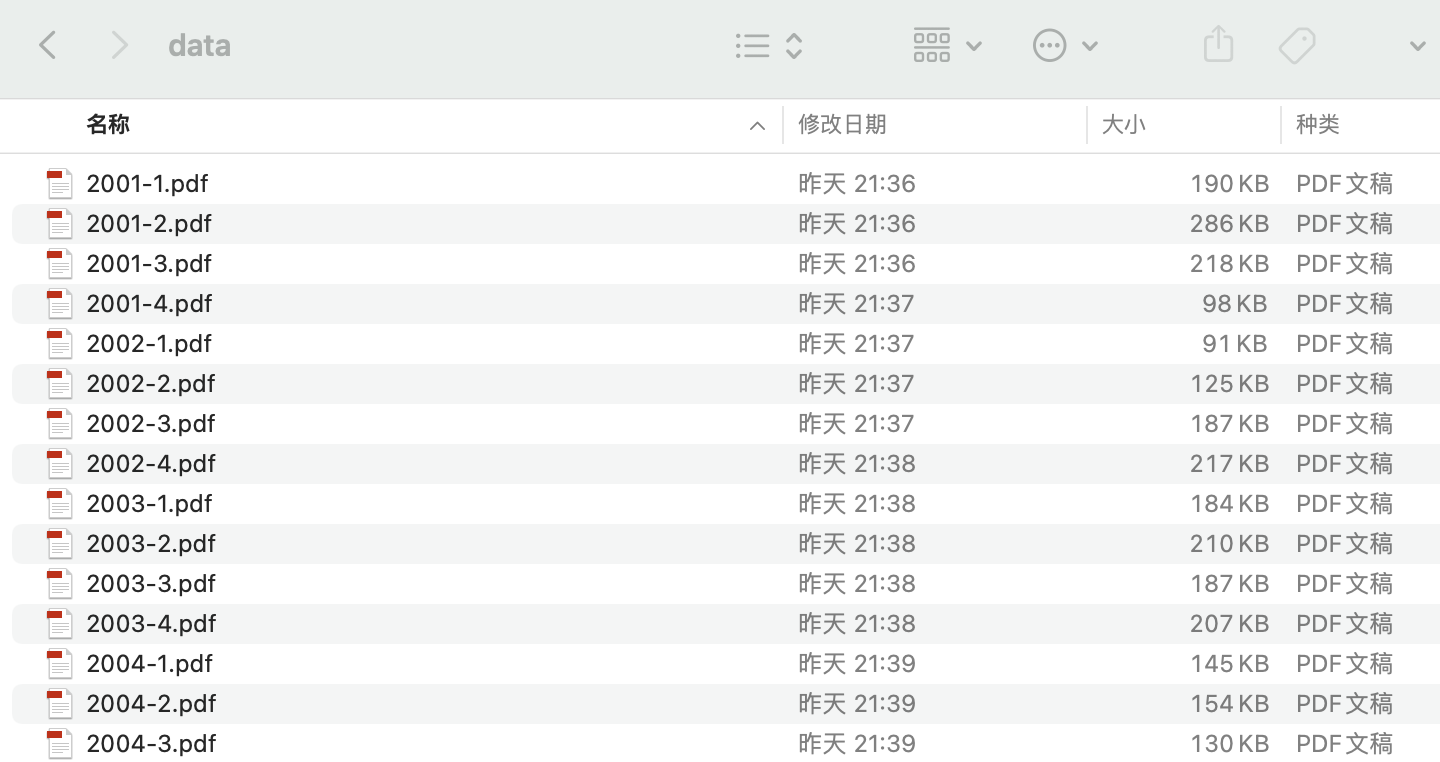import os
import csv

with open('pbc_reports.csv', 'a+', encoding='utf-8', newline='') as csvf:
#年份、季度、报告文本
fieldnames = ['year', 'q', 'text']
writer = csv.DictWriter(csvf, fieldnames=fieldnames)

pdfs = ['data/{}'.format(f) for f in os.listdir('data')]
for pf in pdfs:
data = {
'year':  pf.split('/')[-1][:4],
'q':  pf.split('/')[-1],
}
writer.writerow(data)


## 2. 读取数据#

import pandas as pd
import warnings
warnings.filterwarnings('ignore')



Run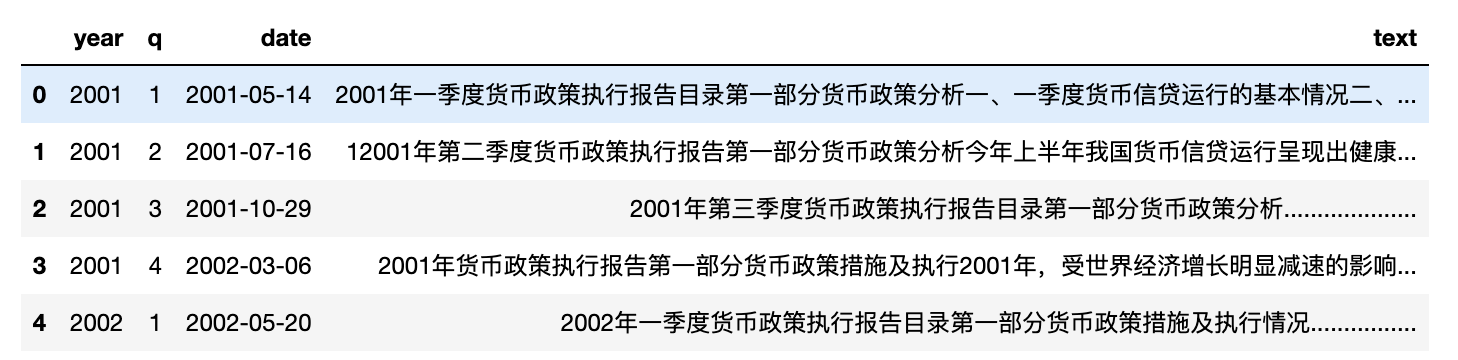#让每一行含有前后两个季度的报告文本
df['text2'] = df['text'].shift(1)
df = df[1:]


Run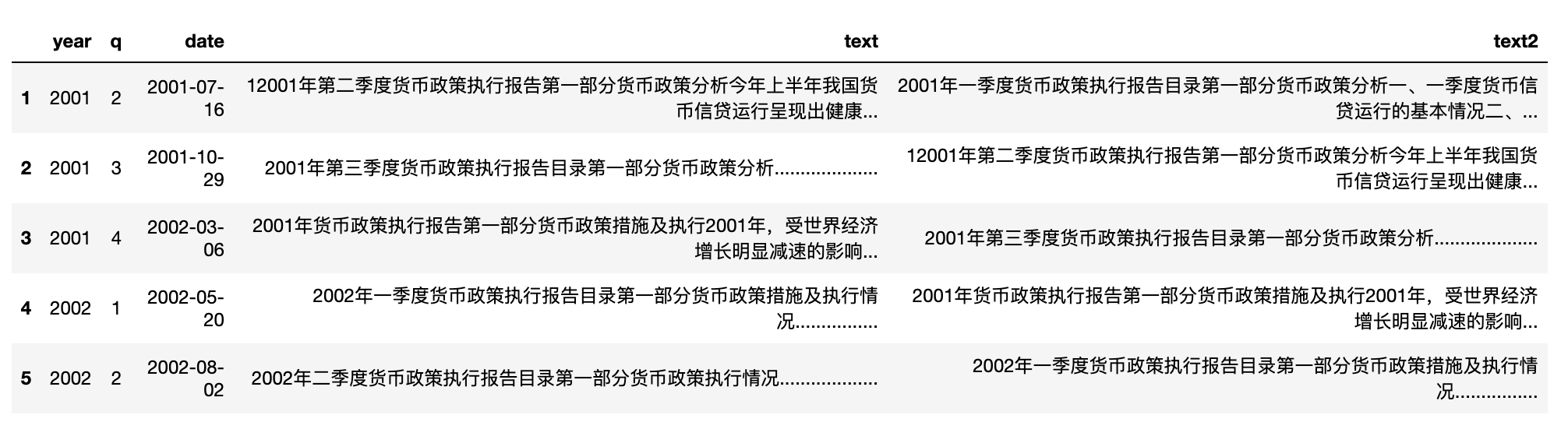## 3. 计算相似度#

import cntext as ct

def cosine_similarity(row):
#row 为 pd.Series 类型数据，类似于字段
try:
sim = ct.cosine_sim(row['text'], row['text2'])
return float(sim)
except:
#异常标记为1
return 1

#计算结果存储到 similarity 字段中
df['similarity'] = df.apply(lambda row: cosine_similarity(row), axis=1)


Run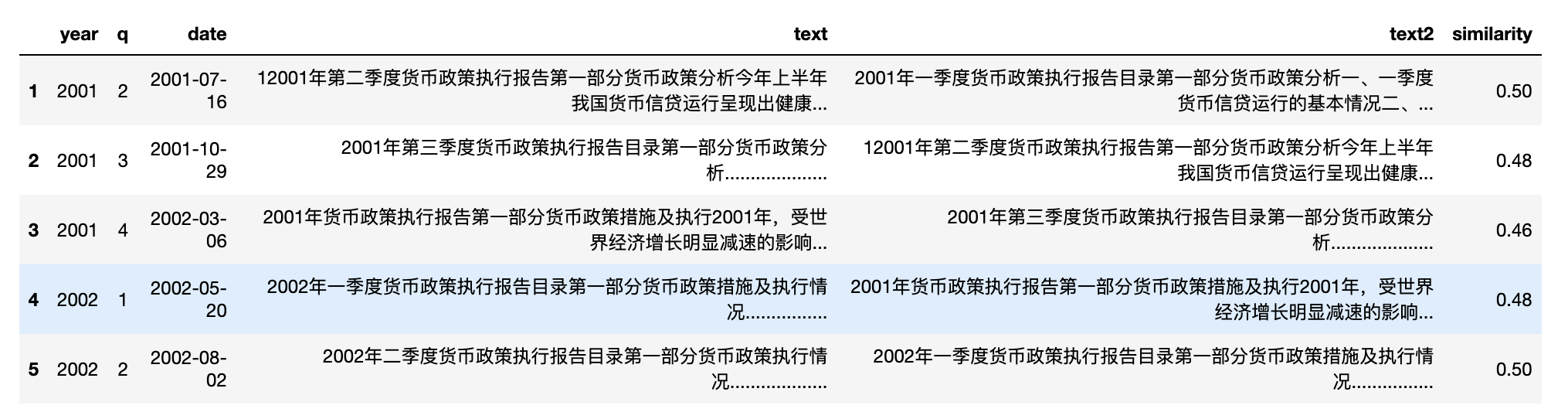## 4. 绘制折线图#

• kind 图表类型，折线图line
• x 横轴字段
• y 纵轴字段
• xlabel 横轴标签
• ylabel 纵轴标签
• title 图标题
import pandas_bokeh

pandas_bokeh.output_notebook()

#选择折线图line
#
df.plot_bokeh(kind = 'line',
x = 'date',
y = 'similarity',
title = '2001~2022央行货币政策相似度趋势',
xlabel = '报告发布日期',
ylabel = '相似度')


Run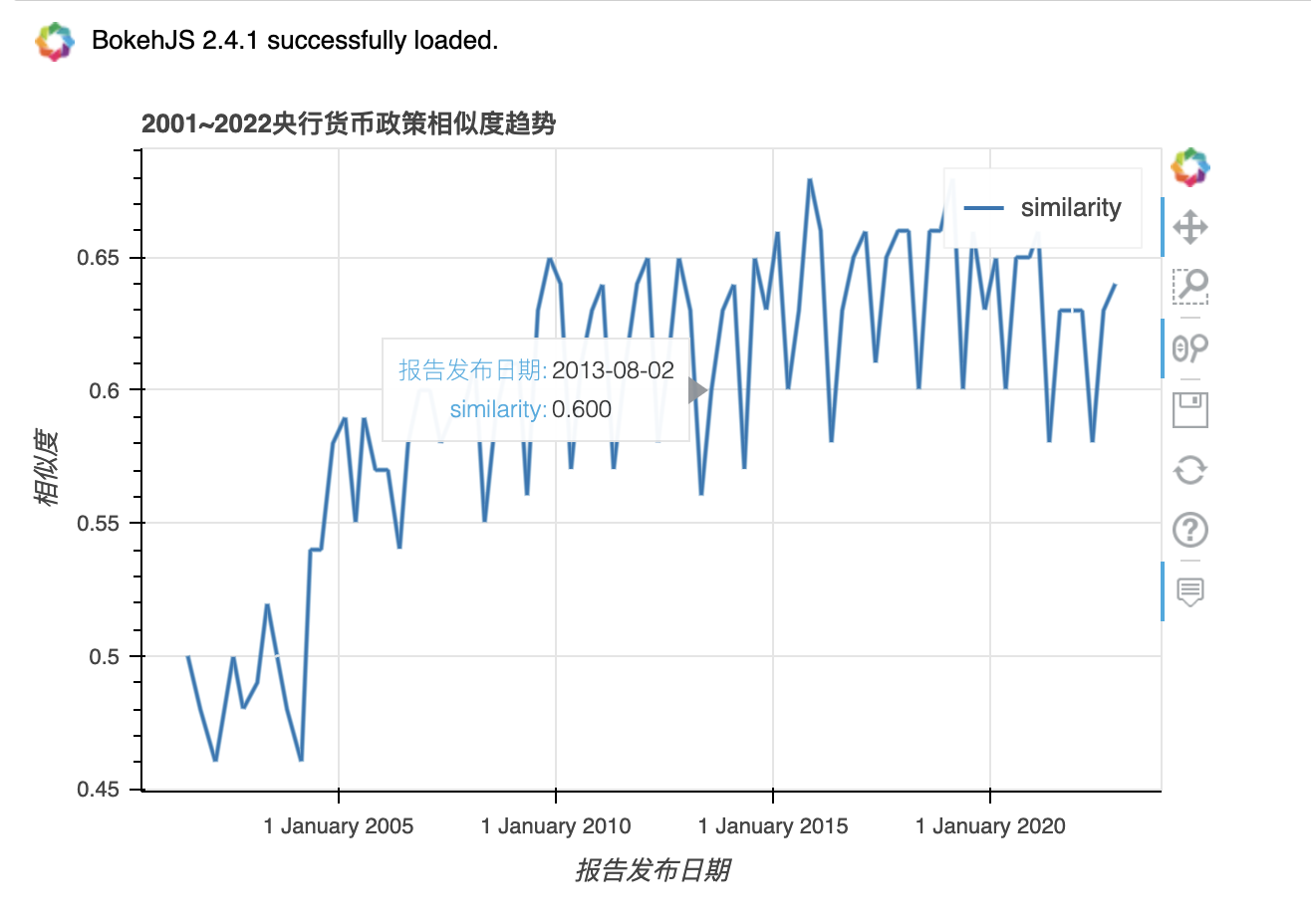import pandas_bokeh

pandas_bokeh.output_notebook()

#三季度移动平均线
df['ma3_similarity'] = df['similarity'].rolling(window=3, center=True, min_periods=1).mean()

df.plot_bokeh(kind = 'line',
x = 'date',
y = 'ma3_similarity',
title = '2001~2022央行货币政策相似度趋势',
xlabel = '报告发布日期',
ylabel = '相似度')


Run